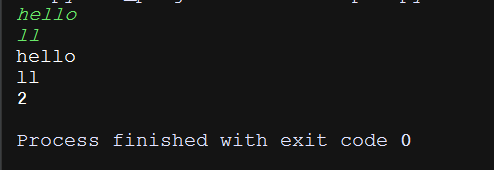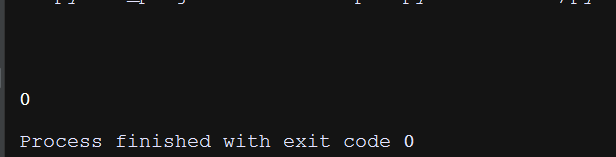# Leetcode题目之求字符串中子串位置指针索引

Implement strStr().

Return the index of the first occurrence of needle in haystack, or -1 if needle is not part of haystack.

Example 1:

Input: haystack = "hello", needle = "ll"
Output: 2


Example 2:

Input: haystack = "aaaaa", needle = "bba"
Output: -1


#-*-Coding:UTF-8-*-
class Solution:
def strStr(self, haystack, needle):
"""
:type haystack: str
:type needle: str
:rtype: int
"""
num = len(needle)
if len(haystack) < num:
return -1
elif haystack == "" and needle == "":
return 0
else:
for i in range(len(haystack)):#0 1 2 3 4
if(haystack[i:num+i]==needle):#[0:2]
return i
return -1
if __name__ == '__main__':
S = Solution()
h = input("")
n = input("")
print(h)
print(n)
print(S.strStr(h,n))我写了两个输出语句用来检测输入是否正确

2018.12.12 1:12am